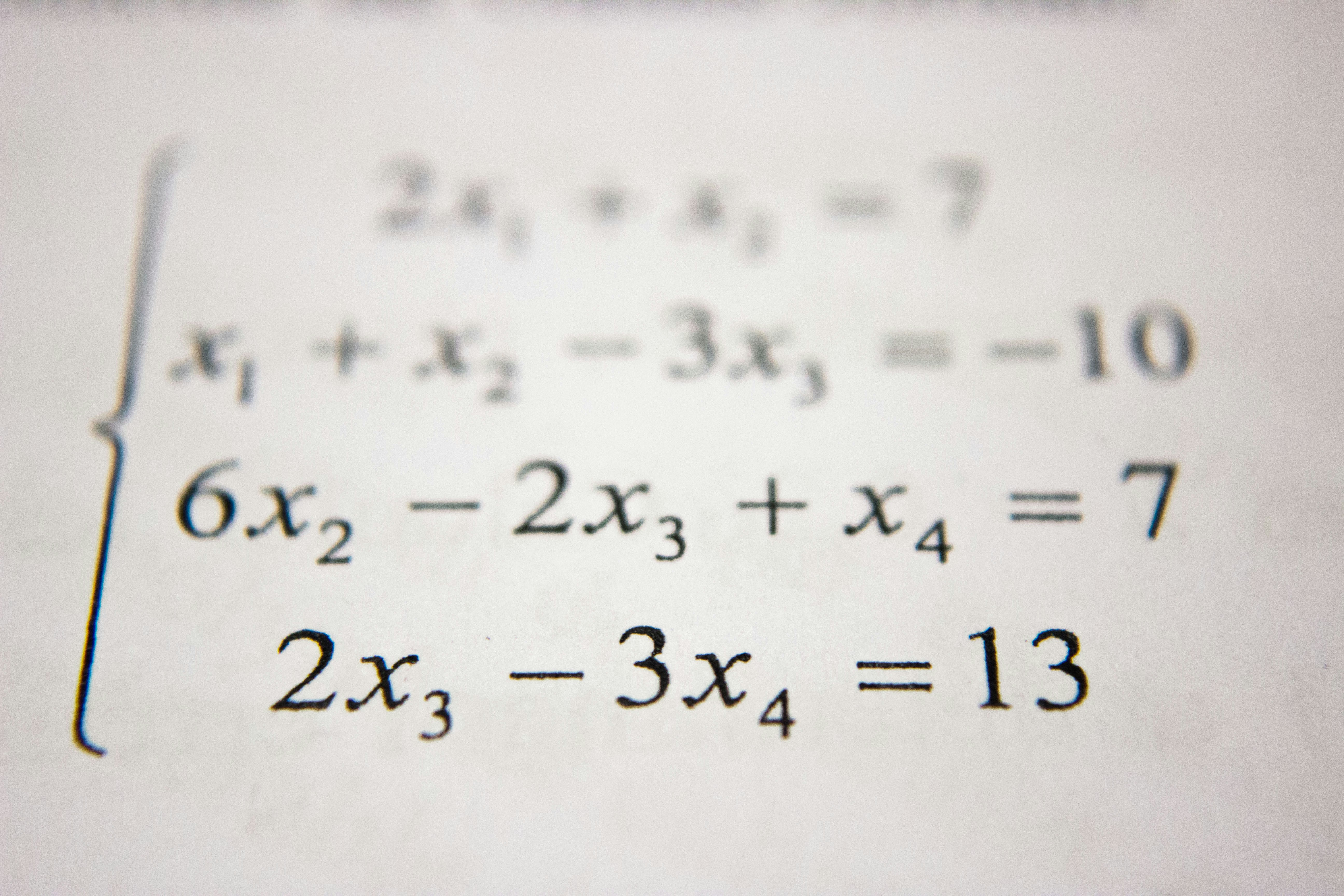Hackerrank - Set .add() solution

# Hackerrank - Set .add() solutionIf we want to add a single element to an existing set, we can use the .add() operation.
It adds the element to the set and returns 'None'.

Example

>>> s = set('HackerRank')
>>> print s
set(['a', 'c', 'e', 'H', 'k', 'n', 'r', 'R'])
None
>>> print s
set(['a', 'c', 'e', 'HackerRank', 'H', 'k', 'n', 'r', 'R'])


Rupal has a huge collection of country stamps. She decided to count the total number of distinct country stamps in her collection. She asked for your help. You pick the stamps one by one from a stack of  country stamps.

Find the total number of distinct country stamps.

Input Format

The first line contains an integer , the total number of country stamps.
The next  lines contains the name of the country where the stamp is from.

Constraints

Output Format

Output the total number of distinct country stamps on a single line.

Sample Input

7
UK
China
USA
France
New Zealand
UK
France


Sample Output

5


Explanation

UK and France repeat twice. Hence, the total number of distinct country stamps is  (five).

### Solution in Python

items = set()
for i in range(int(input())):
print(len(items))